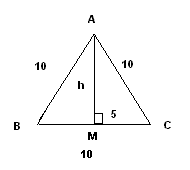# Triangle Problems

Triangle problems are presented along with their detailed solutions.

## Problem 1

The right triangle shown below has an area of 25. Find its hypotenuse.Solution to Problem 1:

• Since the x coordinates of points A and B are equal, segment AB is parallel to the y axis. Since BC is perpendicular to AB then BC is parallel to the x axis and therefore y, the y coordinate of point C is equal to 3. We now need to find the x coordinate x of point C using the area as follows
area = 25 = (1/2) d(A,B) * d(B,C)
d(A,B) = 5
d(B,C) = |x - 2|
• We now substitute d(A,B) and d(B,C) in the area formula above to obtain.
25 = (1/2) (5) |x - 2|
• We solve the above as follows
|x - 2| = 10
x = 12 and x = - 8
• We select x = 12 since point C is to the left of point B and therefore its x coordinate is greater than 2.
• We have the coordinates of point A and C and we can find the hypotenuse using the distance formula.
hypotenuse = d(A,C) = sqrt[ (12 - 2) 2 + (3 - 8) 2 ]
= sqrt(125) = 5 sqrt(5)

## Problem 2

Triangle ABC shown below is inscribed inside a square of side 20 cm. Find the area of the triangleSolution to Problem 2:

• The area is given by
area of triangle = (1/2) base * height
= (1/2)(20)(20) = 200 cm 2

## Problem 3

Find the area of an equilateral triangle that has sides equal to 10 cm.

Solution to Problem 3:

• Let A,B and C be the vertices of the equilateral triangle and M the midpoint of segment BC. Since the triangle is equilateral, AMC is a right triangle. Let us find h the height of the triangle using Pythagorean theorem.h 2 + 5 2 = 10 2
• Solve the above equation for h.
h = 5 sqrt(3) cm
• We now find the area using the formula.
area = (1/2)* base * height = (1/2)(10)(5 sqrt(3))
= 25 sqrt(3) cm 2 = 43.3 cm 2

## Problem 4

An isosceles triangle has angle A 30 degrees greater than angle B. Find all angles of the triangle.

Solution to Problem 4:

• An isosceles triangle has two angles equal in size. In this problem A is greater than B therefore angles B and C are equal in size. Since angle A is 30 greater than angle B then A = B + 30 o. The sum of all angles in a triangle is equal to 180 o.
(B+30) + B + B = 180
• Solve the above equation for B.
B = 50 o
• The sizes of the three angles are
A = B + 30 = 80 o
C = B = 50 o

## Problem 5

Triangle ABC, shown below, has an area of 15 mm 2. Side AC has a length of 6 mm and side AB has a length of 8 mm and angle BAC is obtuse. Find angle BAC to the and find length of side BC.Solution to Problem 5:

• Let the size of angle BAC = t. One of the many formulas for the area triangle is.
area = 15 = (1/2) (AC)(AB) sin(t)
• Solve for sin(t) to obtain.
sin(t) = 30 / (8*6) = 0.625
• Solve for t above and take the solution that gives t obtuse
t = Pi - arcsin(0.625)
• Convert t to degrees to obtain
t (approximately) = 141.3 o
• We now use the cosine rule to calculate the length of side BC
BC 2 = AB 2 + AC 2 - 2(AB)(AC)cos(t)
= 64 + 36 - 2(8)(6)cos(141.3 o)
BC = 13.23 mm.

## More References and Links to

Triangles
Solve Right Triangle Problems
Cosine Law Problems
Geometry Tutorials, Problems and Interactive Applets.